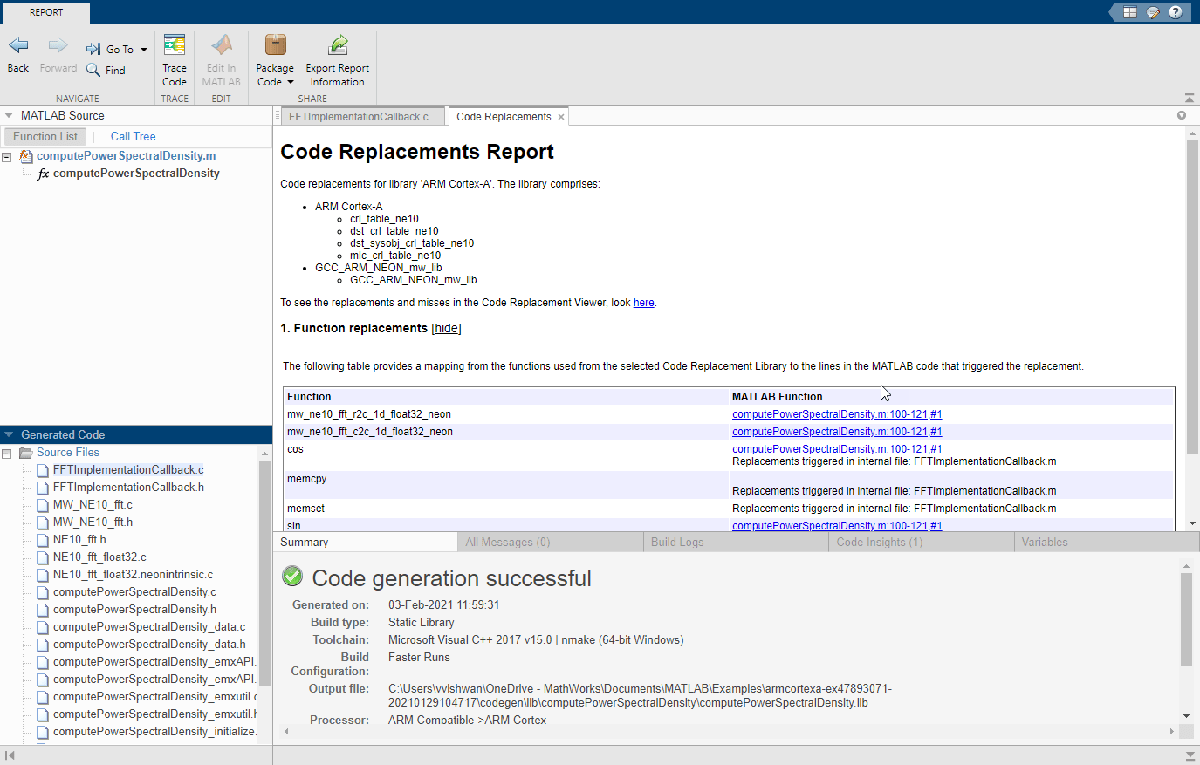FFT for Spectral Analysis on ARM Cortex-A Processor

This example shows how to use Embedded Coder® Support Package for ARM Cortex-A processors to generate optimized C code for the FFT function for spectral analysis with the NE10 library. This example also shows how to use the QEMU ARM Cortex-A9 emulator to verify the generated code. For more details on the FFT function for spectral analysis, see the Fourier Transforms.

Introduction

This example uses the concept of the FFT function for spectral analysis to show how to generate optimized C code for an ARM Cortex-A processor. A common use of FFTs is to find the frequency components of a signal buried in a noisy time-domain signal. For more information, see the fft function.

The MATLAB® function computePowerSpectralDensity computes the power spectral density of the input array signal (noisySignal) for an n-point transform length (nfft). The fft function computes the discrete Fourier transform (DFT) of noisySignal by using a fast Fourier transform (FFT) algorithm. For details about the power spectral density, see the Power Spectral Density Estimates Using FFT.

function Pyy = computePowerSpectralDensity(noisySignal,nfft)
Y = fft(noisySignal,nfft); % Find the FFT of the Noisy Signal
Pyy = Y.*conj(Y)/nfft; % Compute the Power Spectral Density using the complex conjugate
end

Using the Code Replacement Library (CRL), you can generate optimized C code for computing power spectral density function. You can verify the generated code using the Processor-in-the-Loop (PIL) workflow on the QEMU ARM Cortex-A9 emulator. For more information on PIL, see Code Verification Through Software-in-the-Loop and Processor-in-the-Loop Execution. For more information on the QEMU emulator, see the QEMU website.

The Embedded Coder Support Package for ARM Cortex-A processor also supports code generation for these cases:

• Input array signal length (noisySignal) not equal to n-point transform length (nfft)

• Variable-sized input signal

• N-dimensional input signal

• Non-Power-of-2 input signal

Use Case 1 : Non-Power-of-2 Input Signal Code Generation

This use case has an FFT input signal that is of fixed size and equal to the n-point transform length. The length of the input signal is a non-power-of-2. To generate C code, follow these steps.

1. Create an input signal for the FFT calculation.

freq = 1000;       % Sampling frequency
t = 0:1/freq:0.25; % Time axis

data = single(sin(2*pi*50*t) + sin(2*pi*120*t)); % Data has waves of frequency 50Hz & 120Hz

noise = single(2*randn(size(t))); % Noise data

noisySignal = data + noise; % Noisy signal of length 251
nfft = length(noisySignal); % n-point transform of 251

2. Create a code generation configuration object for generating a C/C++ static library.

cfg = coder.config('lib');
cfg.CodeReplacementLibrary = 'ARM Cortex-A';
cfg.GenerateCodeReplacementReport = true;
cfg.TargetLangStandard = 'C89/C90 (ANSI)';
cfg.HardwareImplementation.ProdHWDeviceType = 'ARM Compatible->ARM Cortex';
cfg.GenCodeOnly = true;

3. Generate C code for the MATLAB function computePowerSpectralDensity by using the codegen command.

codegen computePowerSpectralDensity -config cfg -args {noisySignal,nfft} -launchreport

4. Check the report and the generated code. This figure shows the Code replacement report.5. Before verifying the generated code, change the code configuration object to run on a QEMU ARM Cortex-A9 emulator.

cfg.GenCodeOnly = false;
cfg.VerificationMode = 'PIL';
hw = coder.hardware('ARM Cortex-A9 (QEMU)');
cfg.Hardware = hw;
cfg.StackUsageMax = 1024;

6. Verify the generated code with the PIL.

codegen computePowerSpectralDensity -args {noisySignal,nfft} -config cfg -report
yTargetPIL = computePowerSpectralDensity_pil(noisySignal,nfft);

Use Case 2 : Variable Input Signal Code Generation

This use case has an FFT input signal of variable size that uses dynamic memory allocation. To generate C code, follow these steps.

1. Create a code generation configuration object and generate C code for the MATLAB function computePowerSpectralDensity by using the codegen command.

cfg = coder.config('lib');
cfg.CodeReplacementLibrary = 'ARM Cortex-A';
cfg.GenerateCodeReplacementReport = true;
cfg.TargetLangStandard = 'C89/C90 (ANSI)';
cfg.HardwareImplementation.ProdHWDeviceType = 'ARM Compatible->ARM Cortex';
cfg.GenCodeOnly = true;

codegen computePowerSpectralDensity -config cfg -args {coder.typeof(single(1),[1 inf]),coder.typeof(1)} -report

2. Before verifying the generated code, change the code configuration object to run on a QEMU ARM Cortex-A9 emulator. Verify the generated code with the PIL by using a different FFT input signal and n-point transform length.

cfg.GenCodeOnly = false;
cfg.VerificationMode = 'PIL';
hw = coder.hardware('ARM Cortex-A9 (QEMU)');
cfg.Hardware = hw;
cfg.StackUsageMax = 1024;

codegen computePowerSpectralDensity -config cfg -args {coder.typeof(single(1),[1 inf]),coder.typeof(1)} -report
noisySignal = rand(1,167,'single');
nfft = 221;

yTargetPILVarSize = computePowerSpectralDensity_pil(noisySignal,nfft);

Use Case 3 : N-Dimensional Array Input Signal Code Generation

This use case has an input signal that is an N-dimensional array and has an FFT input signal that is not equal to n-point transform length. To generate C code, follow these steps.

1. Create a code generation configuration object and generate C code for the MATLAB function computePowerSpectralDensity for a two-dimensional array input.

cfg = coder.config('lib');
cfg.CodeReplacementLibrary = 'ARM Cortex-A';
cfg.GenerateCodeReplacementReport = true;
cfg.TargetLangStandard = 'C89/C90 (ANSI)';
cfg.HardwareImplementation.ProdHWDeviceType = 'ARM Compatible->ARM Cortex';
cfg.VerificationMode = 'PIL';
hw = coder.hardware('ARM Cortex-A9 (QEMU)');
cfg.Hardware = hw;
cfg.StackUsageMax = 1024;

codegen computePowerSpectralDensity -config cfg -args {coder.typeof(single(1),[100 2]),coder.Constant(100)} -report

2. Verify the generated code with the PIL.

noisySignal = rand(100,2,'single');
nfft = 100;

yTargetPILVarSize1 = computePowerSpectralDensity_pil(noisySignal,nfft);